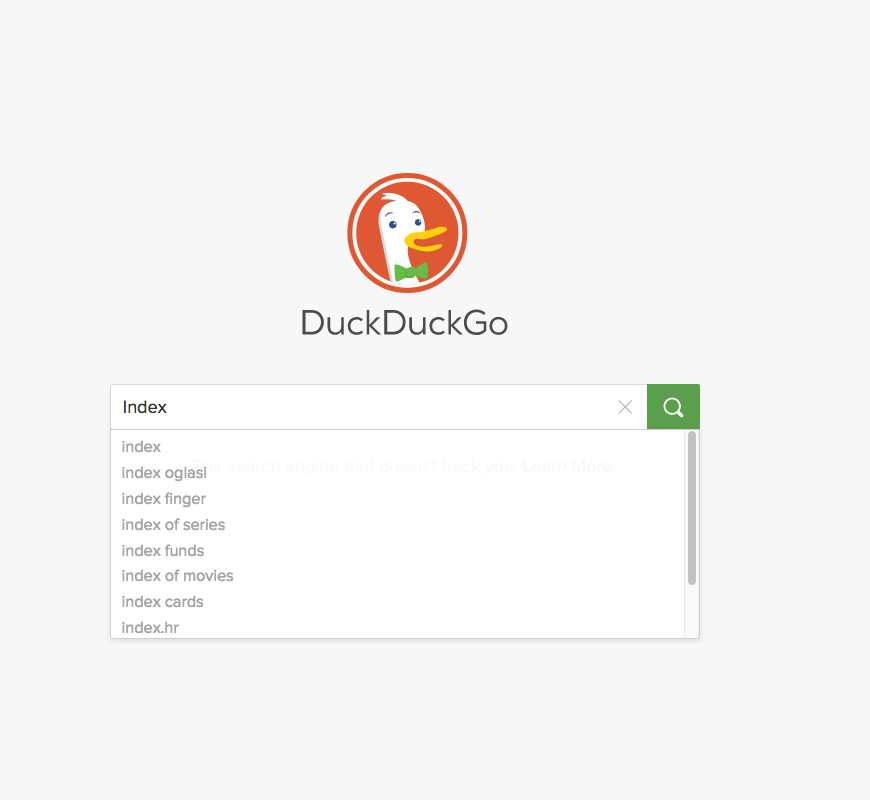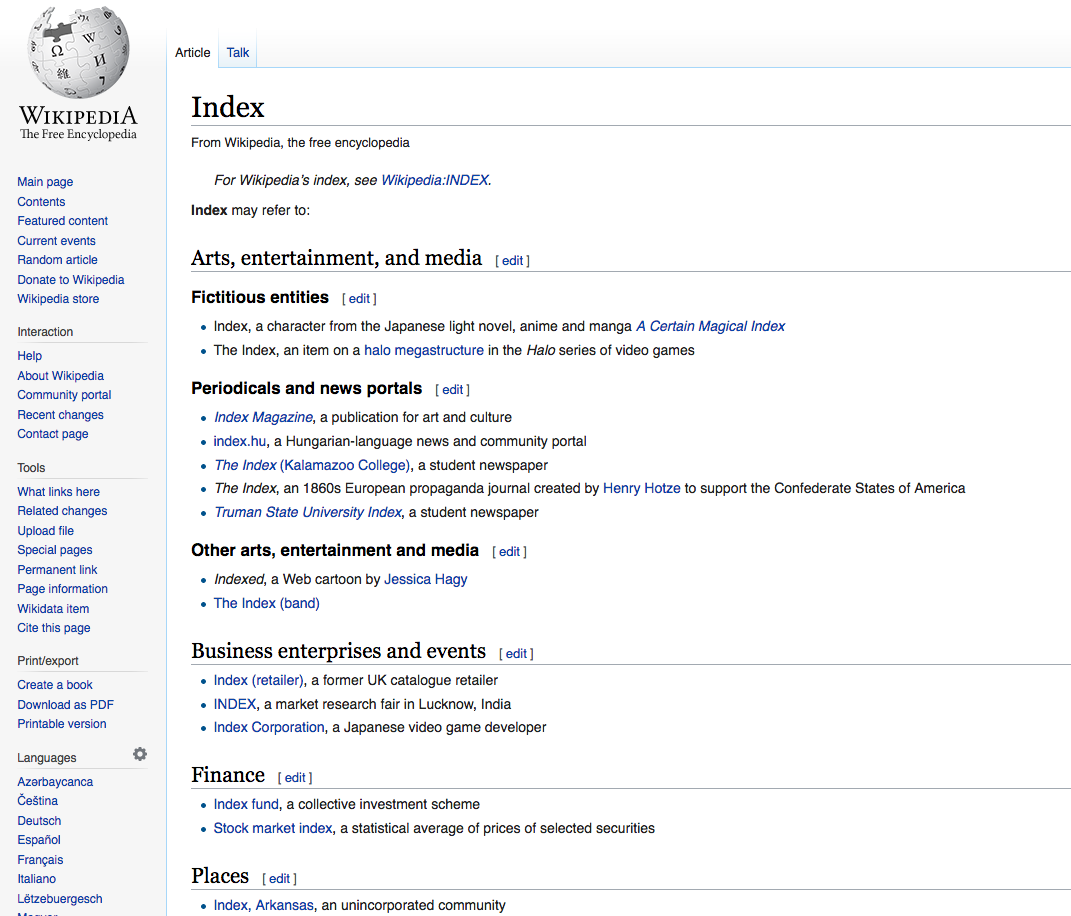CSE-4/562 Spring 2019 - Indexing (Part 2)

### Indexing (Part 2)

#### CSE-4/562 Spring 2019

February 20, 2019

### Index### DataData, even if well organized still requires you to page through a lot.

An index helps you quickly jump to specific data you might be interested in.

### Data Organization

Unordered Heap
No organization at all. $O(N)$ reads.
(Secondary) Index
Index structure over unorganized data. $O(\ll N)$ random reads for some queries.
Clustered (Primary) Index
Index structure over clustered data. $O(\ll N)$ sequential reads for some queries.

### Hash Indexes

A hash function $h(k)$ is ...

... deterministic
The same $k$ always produces the same hash value.
... (pseudo-)random
Different $k$s are unlikely to have the same hash value.

Modulus $h(k)\%N$ gives you a random number in $[0, N)$

### Problems

$N$ is too small
Too many overflow pages (slower reads).
$N$ is too big
Too many normal pages (wasted space).

Idea: Resize the structure as needed

To keep things simple, let's use $$h(k) = k$$

(you wouldn't actually do this in practice)

### Problems

Changing hash functions reallocates everything
Only double/halve the size of a hash function
Changing sizes still requires reading everything
Idea: Only redistribute buckets that are too big

### Dynamic Hashing

• Add a level of indirection (Directory).
• A data page $i$ can store data with $h(k)%2^n=i$ for any $n$.
• Double the size of the directory (almost free) by duplicating existing entries.
• When bucket $i$ fills up, split on the next power of 2.
• Can also merge buckets/halve the directory size.